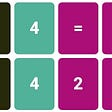# The Monty Hall Problem and The Bayes’ Theorem

The Monty Hall Problem is a mathematical puzzle, loosely based on the TV game show Let's Make a Deal, hosted originally by Monty Hall, that can be solved using the Bayes’ Theorem, an important theorem in the field of statistics and probability theory, hence in the field of Data Science.

Imagine you’re invited into a game show to a chance of winning a fancy new car. The rules are simple: Out of a given number of doors, choose correctly the one where the car stands behind it. In front of you, there are three doors.

You begin by choosing one door. The host, Monty Hall, proceeds to open one of the remaining doors. Monty *never* opens a door where the car is, instead, he always opens a door and reveals that behind it has a goat. After doing so, you’re given the chance to choose from:

a) Open the originally selected door
b) Switch to the other unopened door

Our initial intuition is to think that these options are equiprobable — 50% chance of winning either way — since we are left with the two remaining unopened doors.

In this article, we'll take a look on the demonstration on why the statement above is untrue and how to solve this problem.

# Conditional Probability

Conditional probability is a measure of the probability that an event occurs given that another event has also occurred.

It is often stated as the probability of B given A and is written as P(B|A), where the probability of B depends on that of A happening, and it is given by the fraction of probability B that intersects with A:

If the two events are independent — knowledge about either event does not alter the likelihood of each other — then, the conditional probability of B given A is simply the probability of B, P(B|A) = P(B).

# The Bayes’ Theorem

Bayes’ theorem allows you to update predicted probabilities of an event by incorporating new information.

While conditional probabilities can provide extremely useful information, limited information is often supplied or at hand. Therefore, it can be useful to reverse a condition probability using Bayes’ theorem.

Note that P(A∩B) is the probability of both A and B occurring, which is the same as the probability of A occurring times the probability that B occurs given that A occurred. It is also the same as the probability of B occurring times the probability that A occurs given that B occurred.

If {Bn: n = 1,2,3,…} is a finite or countably infinite partition of Ω, i.e., a set of disjoint events whose union is Ω, then for any event A of the same probability space, the Law of Total Probability gives:

We can see in the image below, that B1, B2, .., B6 are disjoint events, and to access the probability of A, we can sum its intersects with each portion Bn.

Thus, the Bayes’ Theorem gives us the posterior probability — after taking into consideration new information, i.e., the event A occurred — by updating the prior probability, and the denominator can be written using the Law of Total Probability.

# So, should we switch doors?

The Bayes’ Theorem describes the change in probability of a given hypothesis based on new information.

In this case, it’s the probability that our initial guess was the right choice (that is, the chosen door has a car behind it), given that the host showed another door with a goat behind it (that is, one of the remaining door was the wrong choice).

Suppose our initial guess was door number 1, and Monty Hall revealed that behind door number 3 there is a goat.

At first, all doors holds the same probability of having a car behind it, 1/3, as there are only one car and two goats.

Let H be the hypothesis “behind door 1 has a car”, and E be the evidence given by the host that behind door 3 there is a goat. Then, we can calculate the conditional probability P(H|E).

P(H): prior probability that “behind door 1 has a car” = 1/3
P(E|H): probability that behind door 3 there is a goat, given that behind door 1 there is a car. The two events are independent, and the probability that behind door 3 there is a goat is equal to 1, as Monty always opens a door with a goat behind.
P(E): can be written as the sum of the intersect E∩H and E∩Hc, as the union of H and Hc (complementary event) is 1, i.e., Ω.
P(Hc) = 1- P(H) = 1- 1/3 = 2/3

We now have our posterior probability that “behind door 1 has a car”. We used Bayes’ Theorem to update our prior probability using new information given by the host. We before had a 33.3% chance of winning a car choosing door 1, and after Monty opening door 3, the chance is still 33.3%, and not 50% as our intuition would have guide us! That means that our complementary event, “behind door 1 does not has a car”, i.e., “behind door 2 has a car” has probability 66.7%. The numbers are in favor of door number 2!

If you’re still on the fence about the choice, we can look at all the possible scenarios, shown in the table below:

The table shows that in all scenarios, switching to the door offered by the host gives us a 2/3 chance of winning, while staying at the originally selected door gives us a 1/3 chance of winning.

Another way to visualize this is to take the possible outcomes of door number 1 and the remaining pair (door 2, door 3).

There is a chance of 1/3 that door 1 has a car, and 2/3 there is a goat, so it’s more likely we’ll get a goat in the beginning. On the other hand, looking at the pair (door 2, door 3), the chance of the outcome (goat, car) is 2/3 and the outcome (goat, goat) is 1/3, so it’s more likely that the car is on either door 2 or door 3.

When Monty opens door number 3, those same probabilities are remained true: door 1 has a car behind it with probability 1/3, while one of (door 2, door 3) has a car behind it with probability 2/3, and since we know that the car is not behind door 3, this probability is now of door 2 alone.

# Conclusion

tl;dr: We should switch doors!

Hopefully, this article helped on the intuition behind this problem, and you can now explain with confidence, the best option to follow. :-)

# Bonus

This puzzle can be seen in season 1, episode 5 of the Netflix series Alice in Borderland「今際の国のアリス」, a Japanese science fiction suspense-thriller drama based on the manga of the same name.

--

--

--

## More from Juliana Daikawa

Love podcasts or audiobooks? Learn on the go with our new app.

## Understanding the need for Mathematics## A Compact Version of Riemann Prime Counting Function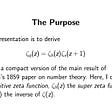## The least informative pulse## Exploring the Dot & Cross Product.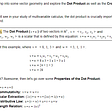## The formula of the Universe is for suckers!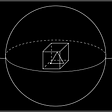## Spinning Around In Cycles With Directed Acyclic Graphs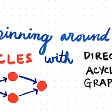## The math of the Flat Earth…## Destroying Wordle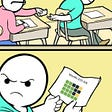## Ordinary Least Squares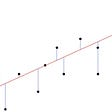## Why do you even need Statistical Distributions in Layman’s terms?## Some Mathematical Analysis on Nerdle Game